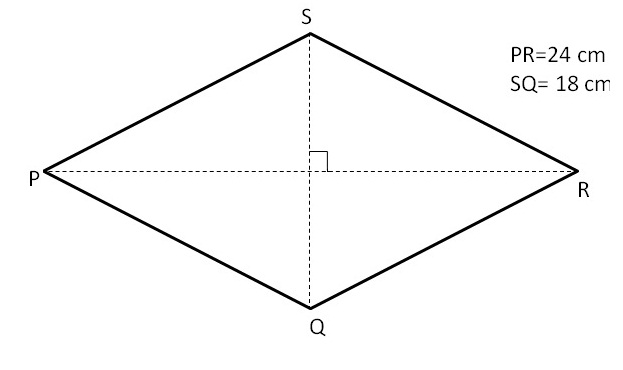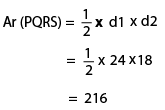Area of rhombus

## Objective

To show that the area of rhombus is half the product of its diagonals.

### Theory

1. A rhombus is a simple (non-self-intersecting) quadrilateral whose all four sides are of same length.
2. If a parallelogram has two consecutive sides congruent, it is a rhombus.
3. If two triangles are congruent then their areas are equal.
4. Area of a triangle = 1/2 X base X height
5. Area of a rectangle = Length X Breadth
6. Diagonals of rhombus are perpendicular to each other.

##In above figure EHGF is rhombus with diagonal HF (length d1) and diagonal EG (length d2)

Area of rhombus EHGF = Area of triangle EFH + Area of triangle FHG

= half of the product of the diagonals

### Example

Find the area of the following rhombus.### Solution:

In the given figure,

PR = d1= 24 cm.

SQ = d2 = 18 cm.So, the area of the rhombus PQRS is 216 cm2.